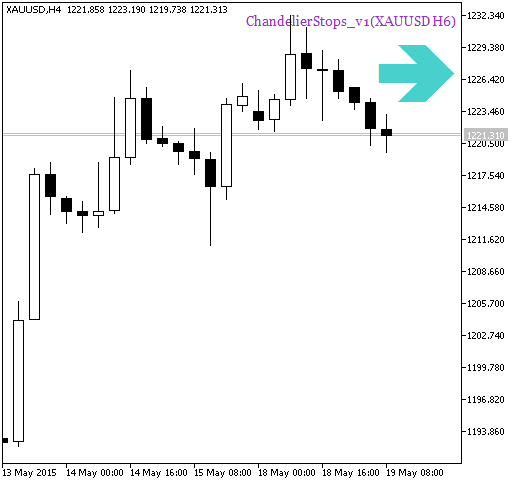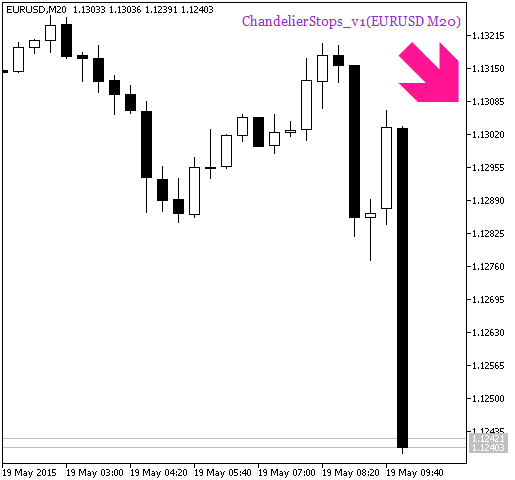627

2015.07.24 13:26

2016.11.22 07:33

1. ChandelierStops_v1 指标的输入参数:
```input string Symbol_="";                               // 金融工具
input ENUM_TIMEFRAMES Timeframe=PERIOD_H6;             // 指标计算的时间帧
input uint   Length=20;
input uint   ATRPeriod=10;
input double Kv=3;
```

2. ChandelierStops_v1_HTF_Signal 指标所需要的可视化输入参数:
```//---- 指标显示设置
input uint SignalBar=0;                                // 获取信号的柱线号码 (0 - 当前柱线)
input string Symbols_Sirname=INDICATOR_NAME"_Label_";  // 指标标签名
input color Upsymbol_Color=clrMediumTurquoise;         // 上涨符号颜色
input color Dnsymbol_Color=clrDeepPink;                // 下跌符号颜色
input color IndName_Color=clrDarkOrchid;               // 指标名颜色
input uint Symbols_Size=60;                            // 信号符号大小
input uint Font_Size=10;                               // 指标名字体大小
input int X_1=5;                                       // 水平名称位移
input int Y_1=-15;                                     // 垂直名称位移
input bool ShowIndName=true;                           // 显示指标名
input ENUM_BASE_CORNER  WhatCorner=CORNER_RIGHT_UPPER; // 坐落边角
input uint X_=0;                                       // 水平位移
input uint Y_=20;                                      // 垂直位移
```

3. ChandelierStops_v1_HTF_Signal 指标触发提示和声音信号所需的输入参数:
```//---- 提示设置
```ChandelExit_HTF_SignalChandelExitSignRainbow_HMARainbow_HMA_HTF